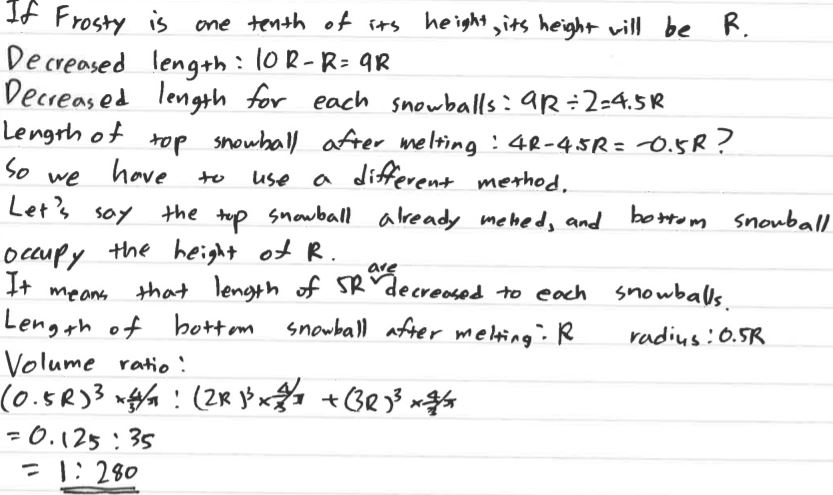# Frosty Is Melting!

##### Age 14 to 16Challenge Level

When Frosty is half his initial height, show that the ratio of his volume to his initial volume is 37 : 224

Ziyao from Garden International School in Malaysia, Jaeuk from Brighton College Abu Dhabi in the UAE and Callum from Ilkley Grammar school in the UK used a similar method to solve this problem. This is Ziyao's work:

Note that the formula for the volume of a sphere will come in very handy: $\frac43\pi r^3$

In the first question, Frosty has melted to half of his original height. It is given that the radius of the snowballs decreases by the same amount, so to get the radius of the spheres at half height we do the following below:

The height is $2R\times2+3R\times2=10R.$  $10R\div2=5R$ and so the height has decreased by $5R.$ Therefore each sphere’s diameter has decreased by $5R\div2=2.5R$ according to the information in the quotation. The radii of the spheres after the height has decreased by $\frac12$ will be $\frac{4R - 2.5R}2=0.75R,$ $\frac{6R - 2.5R}2=1.75R.$

Calculating the volumes

The original volume [of the smaller snowball] is $8R^3\times\frac43\times\pi=32R^3\frac\pi3$ (applying the formula for the volume of a sphere)
[The original volume of the larger snowball is] $27R^3\times\frac43\times\pi=36R^3\pi$
$\dfrac{32R^3\pi}3+\dfrac{108R^3\pi}3=\dfrac{140R^3\pi}3$

The volume when the height has decreased by $\frac12$:
$\left(\frac34R\right)^3\times\frac43\times\pi=1\times\left(\frac34\right)^2R^3\times\pi=\dfrac{9R^3\pi}{16},$ (again, applying the formula for the volume of a sphere)
$\left(\frac74R\right)^3\times\frac43\times\pi=\dfrac{343R^3\pi}{64}\times\frac43\times\pi=\dfrac{343R^3\pi}{48},$
and $\dfrac{9R^3\pi}{16}+\dfrac{343R^3\pi}{48}=\dfrac{27R^3\pi}{48}+\dfrac{343R^3\pi}{48}=\dfrac{370R^3\pi}{48}$

The final ratio

The ratio is $\dfrac{370R^3\pi}{48}:\dfrac{140R^3\pi}3$
multiply both sides by $48$ to get $370R^3\pi:2240R^3\pi$
divide both sides by $R^3\pi$ to get $370:2240$
and finally, divide by $10$ on both sides to get $37:224$

J from EIGCDN in Switzerland and Yonwoo solved the problem by writing a formula for Frosty's height. This is J's work:

The initial height of the snowman is $2\times2R+2\times3R$ (sum of diameters) which is equal to $10R.$

To find the initial volume, we simply substitute the values $2R$ and $3R$ into the general formula:

$\frac43\pi(2R)^3+\frac43\pi(3R)^3=\frac43\pi(8R^3+27R^3)=\frac43\pi(35R^3)=\frac{140}3\pi R^3$

$5R$ is half of the initial snowman's height. Let $D$ be the amount each radius decreases by every minute. After $x$ minutes, the top snowball has a radius of $2R-xD$ and the bottom one has a radius of $3R-xD.$

Using these facts, we can create a formula for the height of the snowman after $x$ minutes:

$2\times(2R-xD)+2\times(3R-xD)=4R-2xD+6R-2xD=10R-4xD$

At the point where the total height is $5R,$ we get $10R-4xD=5R\Rightarrow4xD=5R\Rightarrow xD=\frac54R.$ By plugging this into the expressions for the top and bottom radii, we get:

Top radius $=2R-\frac54R=\frac34R$
Bottom radius $=3R-\frac54R=\frac74R$

Next, we can find the volumes of the snowballs.

From here, J's solution is the same as Ziyao's solution above.

What is this ratio when Frosty is one-tenth of his initial height?

J continued using the same formula, but it led to some very dangerous maths:

So far, we have built up quite a few formulae; now, we can finally apply them.

The snowman's height is $R$ when the height reaches one-tenth of the initial height, $10R.$ Remember that the formula for the height after $x$ minutes is $10R-4xD$ so $10R-4xD=R\Rightarrow4xD=9R\Rightarrow xD=\frac94R.$

We can now substitute into the formulae for top / bottom radii:

Top radius $=2R-\frac94R=-\frac14R$
Bottom radius $=3R-\frac94R=\frac34R$

This part seems a little weird. Negative magnitudes are nonexistent. However, we can proceed because the volume of the bottom snowball is greater than the top radius and so the total volume is still positive. Also note that in this scenario, the top snowball has already melted.

The total volume is:

$\frac43\pi\left(-\frac1{64}R^3+\frac{27}{64}R^3\right)=\frac43\pi\left(\frac{13}{32}R^3\right)=\frac{52}{96}\pi R^3=\frac{13}{24}\pi R^3$

As usual, we ignore $\pi R^3$ and focus on the other coefficient. We compare $\frac{13}{24}$ and $\frac{140}{3}$; by scaling them up to the same denominator, we get $\frac{13}{24}$ and $\frac{1120}{24}.$ The ratio therefore is $13 : 1120$ which cannot be simplified further.

Jaeuk and Yonwoo also realised that the smaller snowball would have melted completely, and they adjusted their calculations to get correct answers. This is Jaeuk's work (click here to see a larger version):